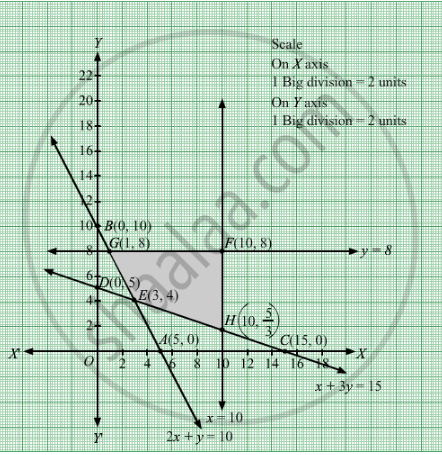# Minimize Z = 5x + 3y Subject to 2 X + Y ≥ 10 X + 3 Y ≥ 15 X ≤ 10 Y ≤ 8 X , Y ≥ 0 - Mathematics

Sum

Minimize Z = 5x + 3y
Subject to

$2x + y \geq 10$
$x + 3y \geq 15$
$x \leq 10$
$y \leq 8$
$x, y \geq 0$

#### Solution

First, we will convert the given inequations into equations, we obtain the following equations:
2x + y = 10, x + 3y = 15, x = 10, y = 8

Region represented by 2x + y ≥ 10:
The line 2x + y = 10 meets the coordinate axes at A(5, 0) and B(0, 10) respectively. By joining these points we obtain the line 2x + y = 10.
Clearly (0,0) does not satisfies the inequation 2x + y ≥ 10. So,the region in xy plane which does not contain the origin represents the solution set of the inequation 2x + y≥ 10.

Region represented by x + 3y ≥ 15:
The line x + 3y = 15 meets the coordinate axes at C(15, 0) and D(0, 5) respectively. By joining these points we obtain the line x + 3y = 15.
Clearly (0,0) satisfies the inequation x + 3y ≥ 15. o,the region in xy plane which does not contain the origin represents the solution set of the inequation x + 3y ≥ 15.

The line x = 10 is the line that passes through the point (10, 0) and is parallel to Yaxis.≤ 10 is the region to the left of the line x = 10.

The line y = 8 is the line that passes through the point (0, 8) and is parallel to X axis.≤ 8 is the region below the line y = 8.

Region represented by x ≥ 0 and y ≥ 0:
Since, every point in the first quadrant satisfies these inequations. So, the first quadrant is the region represented by the inequations x ≥ 0 and ≥ 0.

The feasible region determined by the system of constraints, 2x + y ≥ 10, x + 3y ≥ 15, x ≤ 10, ≤ 8, x ≥ 0 and y ≥ 0 are as follows.The corner points of the feasible region are E(3, 4), $H\left( 10, \frac{5}{3} \right)$ F(10, 8) and G(1, 8).

The values of Z at these corner points are as follows
 Corner point Z = 5x + 3y E(3, 4) 5 × 3 + 3 × 4 = 27 $H\left( 10, \frac{5}{3} \right)$ 5 × 10 + 3× $\frac{5}{3}$ = 55 F(10, 8) 5 × 10 + 3 × 8 = 74 G(1, 8) 5 × 1 + 3 ×  8 = 29
Therefore, the minimum value of Z is 27 at the point F(3, 4). Hence, x = 3 and y =4 is the optimal solution of the given LPP.
Thus, the optimal value of Z is 27.
Concept: Graphical Method of Solving Linear Programming Problems
Is there an error in this question or solution?

#### APPEARS IN

RD Sharma Class 12 Maths
Chapter 30 Linear programming
Exercise 30.2 | Q 11 | Page 32